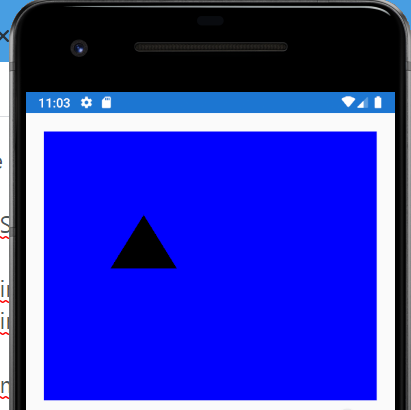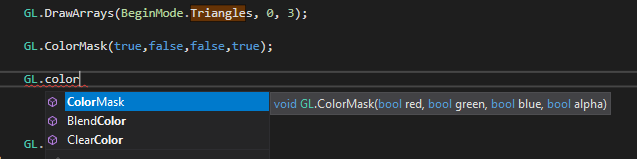## Announcement:

The Xamarin Forums have officially moved to the new Microsoft Q&A experience. Microsoft Q&A is the home for technical questions and answers at across all products at Microsoft now including Xamarin!

To create new threads and ask questions head over to Microsoft Q&A for .NET and get involved today.

# Setting GL.Ortho and Fragment Shader Colours with OpenTK.Graphics.ES30

Could I please ask for advice on the following problem?

I am using Visual Studio 2019, have a Xamarin forms project up and running in the Android Emulator. It animates a triangle by translating it down the screen. Here's the salient code:

My triangle:

```_private float[] m_vertex_buffer_data = { 0.2f, 0.5f, 0.1f,
0.4f, 0.5f, 0.1f,
0.3f,  0.7f, 0.1f };_
```

An update function:

```_private void OffsetTriangle(float offset)
{
m_vertex_buffer_data += offset;
m_vertex_buffer_data += offset;
m_vertex_buffer_data += offset;

GL.BindBuffer(BufferTarget.ArrayBuffer, m_nVertexBuffer);

GL.BufferSubData(BufferTarget.ArrayBuffer, IntPtr.Zero, new IntPtr(9 * sizeof(float)), m_vertex_buffer_data);
}_
```

My shaders are trivial:

```    private void SetShaderSource()
{
m_szVertexShader = "gl_Position = ftransform();";
m_szFragmentShader = "gl_FragColor = vec4(1,0,1,1);";
}
```

My MainPage code is tiny:

``` _public MainPage()
{
Title = "OpenGL";
var view = new OpenGLView { HasRenderLoop = true };

view.HeightRequest = 300;
view.WidthRequest = 300;

GL.Viewport(0, 0, 300, 300);

OffsetTriangle(0.4f);

view.OnDisplay = r =>
{
if(!m_bOGLParametersSet) // I do this once, on the first render
{
CreateShader();

m_bOGLParametersSet = true;

GL.UseProgram(m_nProgram);

GL.GenBuffers(1, out m_nVertexBuffer);

GL.BindBuffer(BufferTarget.ArrayBuffer, m_nVertexBuffer);

GL.BufferData(BufferTarget.ArrayBuffer, new IntPtr(9 * sizeof(float)), m_vertex_buffer_data, BufferUsage.StaticDraw);
}

GL.ClearColor(0.0f, 0.0f, 1.0f, 1.0f);
GL.Clear((ClearBufferMask.ColorBufferBit | ClearBufferMask.DepthBufferBit));

OffsetTriangle(-0.01f);

GL.EnableVertexAttribArray(0);
GL.BindBuffer(BufferTarget.ArrayBuffer, m_nVertexBuffer);

GL.VertexAttribPointer(0, 3, VertexAttribPointerType.Float, false, 0, 0);

GL.DrawArrays(BeginMode.Triangles, 0, 3);

GL.DisableVertexAttribArray(0);
};_
```

And here's the CreateShader function, which as you can see above is called on the first render:

```   private void CreateShader()
{
SetShaderSource();

int nVertexShaderSourceLength = m_szVertexShader.Length;
int nFragmentShaderLength = m_szFragmentShader.Length;

m_nVertexShaderHandle = GL.CreateShader(ShaderType.VertexShader);
m_nFragmentShaderHandle = GL.CreateShader(ShaderType.FragmentShader);

GL.ShaderSource(m_nVertexShaderHandle, m_szVertexShader);
GL.ShaderSource(m_nFragmentShaderHandle, m_szFragmentShader);

GL.CompileShader(m_nVertexShaderHandle);
GL.CompileShader(m_nFragmentShaderHandle);

m_nProgram = GL.CreateProgram();

GL.AttachShader(m_nProgram, m_nVertexShaderHandle);
GL.AttachShader(m_nProgram, m_nFragmentShaderHandle);

GL.LinkProgram(m_nProgram);
}
```

It runs fine, the triangle animates down the screen, here's a screenshot part way through the animation:My questions are:

1) Why is the triangle black, when the fragment shader is setting gl_FragColor = vec4(1,0,1,1); Nothing I do in the fragment shader changes this.

2) I am using OpenTK.Graphics.ES30, the GL class has no Ortho method, so how am I to set the extents of the OpenGL view?
I see that GL.Ortho is available if I use OpenTK.Graphics.ES10 but then many of the other GL functions I use are not available,
in particular all the shader functions.

Thanks for any help,
Mitch.

Tagged:

## Best Answer

• The peoblem turned out to be my shaders. They did not comform the the specification. I changed them to:

m_szVertexShader = "attribute vec3 a_position;" +
"void main(void)" +
"{" +
"gl_Position = vec4(a_position, 1.0);" +
"}";

```        m_szFragmentShader = "precision mediump float;" +
"void main(void)" +
"{" +
"gl_FragColor = vec4(1.0,0.0,0.0,1.0);" +
"}";
```

And all started to work. Thanks very much for your replies.

MItch.

## Answers

• Just wanted to clarify that my shader code is actually:

```private void SetShaderSource()
{
m_szVertexShader = "void main()" +
"{" +
"gl_Position = ftransform();" +
"}";

m_szFragmentShader = "void main()" +
"{" +
"gl_FragColor = vec4(1.0,0.0,1.0,1.0);" +
"}";
}
```
• Member, Xamarin Team Xamurai

1) Why is the triangle black, when the fragment shader is setting gl_FragColor = vec4(1,0,1,1); Nothing I do in the fragment shader changes this.

You can check the following fuctions of `GL`.For example, when I changed to the following code:

```            GL.DrawArrays(BeginMode.Triangles, 0, 3);

GL.ColorMask(true,false,false,true);

GL.DisableVertexAttribArray(0);
```

The triangle color will change to Sky blue.

• Member, Xamarin Team Xamurai

Hi @gnitsuk，have you resolved your question?

• The peoblem turned out to be my shaders. They did not comform the the specification. I changed them to:

m_szVertexShader = "attribute vec3 a_position;" +
"void main(void)" +
"{" +
"gl_Position = vec4(a_position, 1.0);" +
"}";

```        m_szFragmentShader = "precision mediump float;" +
"void main(void)" +
"{" +
"gl_FragColor = vec4(1.0,0.0,0.0,1.0);" +
"}";
```

And all started to work. Thanks very much for your replies.

MItch.

Sign In or Register to comment.